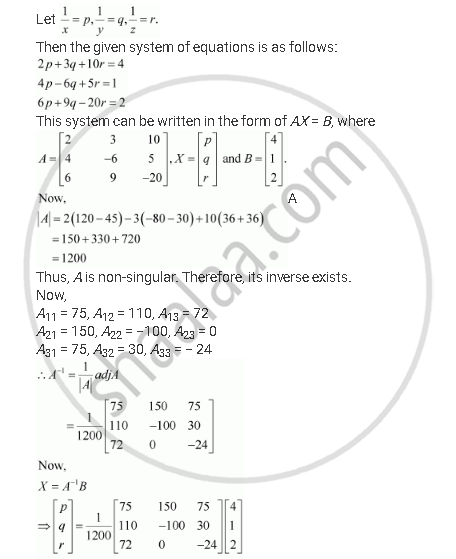Share

# Solve the System of the Following Equations 2/X+3/Y+10/Z = 4 and 4/X-6/Y + 5/Z = 1 and 6/X + 9/Y - 20/X = 2 - CBSE (Science) Class 12 - Mathematics

ConceptApplications of Determinants and Matrices

#### Question

Solve the system of the following equations

2/x+3/y+10/z = 4

4/x-6/y + 5/z = 1

6/x + 9/y - 20/x = 2

#### SolutionIs there an error in this question or solution?

#### Video TutorialsVIEW ALL 

Solution Solve the System of the Following Equations 2/X+3/Y+10/Z = 4 and 4/X-6/Y + 5/Z = 1 and 6/X + 9/Y - 20/X = 2 Concept: Applications of Determinants and Matrices.
S